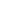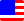Documents  >  Tutorial  >  Operators
Operators

An operator is a symbol that specifies the operation to be performed on an instance. The operator performs an operation on the operand and returns an instance of the result.

Priority

Change priority

Priority

Operators have priorities. Expressions that contain multiple operators are computed from operators with higher priorities.

The following is a list of operators. The smaller number is the higher priority. If operators with the same priority are adjacent, the operation is executed in order from the left of the expression.

 Priority 1 () x(y) Method call operator new new int() new operator this this this operator [] x[y] Indexer operator Priority 2 . x.y Dot operator ++ x++ Postfix ++ operator -- x-- Postfix -- operator Priority 3 + +x Unary + operator - -x Unary - operator ! !x Logical negation operator ++ ++x Prefix ++ operator -- --x Prefix -- operator Priority 4 * x * y Multiplication operator / x / y Division operator % x % y Modulo operator Priority 5 + x + y Addition operator - x - y Subtraction operator Priority 6 < x < y Less than operator <= x <= y Less than or equal operator > x > y Greater than operator >= x >= y Greater than equal operator Priority 7 == x == y Value equality operator != x != y Value inequality operator \$\$ x \$\$ y Reference equality operator !\$ x !\$ y Reference inequality operator Priority 8 & x & y Logical AND operator Priority 9 | x | y Logical OR operator

Change priority

Use parentheses to change the priority of operations. Expressions in parentheses are preferentially operated.

int i = (2 + 2) * 2; // 8